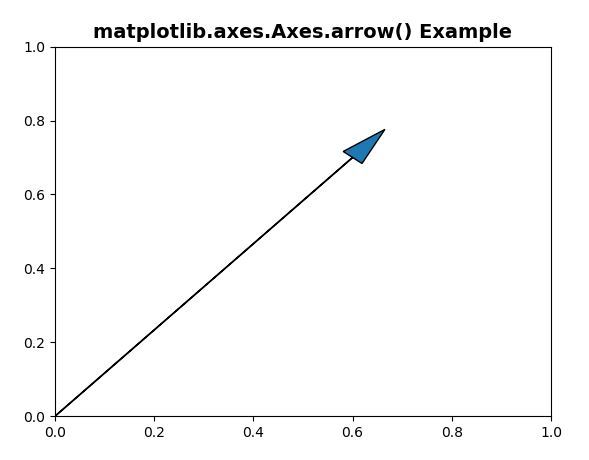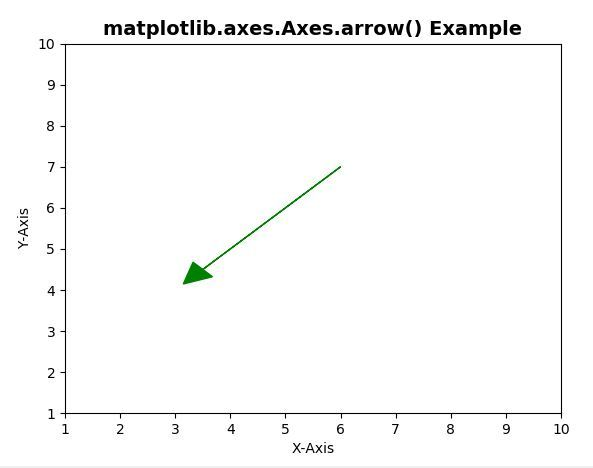Related Articles
Matplotlib.axes.Axes.arrow() in Python
• Last Updated : 13 Apr, 2020

Matplotlib is a library in Python and it is numerical – mathematical extension for NumPy library. The Axes Class contains most of the figure elements: Axis, Tick, Line2D, Text, Polygon, etc., and sets the coordinate system. And the instances of Axes supports callbacks through a callbacks attribute.

## matplotlib.axes.Axes.arrow() Function

The Axes.arrow() function in axes module of matplotlib library is also used to add an arrow to the axes. This function is used to draws an arrow from (x, y) to (x+dx, y+dy)

Syntax:

```Axes.arrow(self, x, y, dx, dy, **kwargs)
```

Parameters: This method accept the following parameters that are described below:

• x, y: These parameter are the x and y coordinates of the arrow base.
• dx, dy: These parameter are the length of the arrow along x and y direction.

Returns: This method returns the arrow which is a created FancyArrow object.

Below examples illustrate the matplotlib.axes.Axes.arrow() function in matplotlib.axes:

Example-1:

 `# Implementation of matplotlib function``import` `matplotlib.pyplot as plt`` ` `ax ``=` `plt.axes()``ax.arrow(``0``, ``0``, ``0.6``, ``0.7``, head_width ``=` `0.05``, ``         ``head_length ``=` `0.1``)`` ` `ax.set_title(``'matplotlib.axes.Axes.arrow() Example'``, ``             ``fontsize ``=` `14``, fontweight ``=``'bold'``)`` ` `plt.show()`

Output:Example-2:

 `# Implementation of matplotlib function``import` `matplotlib.pyplot as plt`` ` `ax ``=` `plt.axes()``ax.arrow(``6``, ``7``, ``-``2.5``, ``-``2.5``, head_width ``=` `0.5``,``         ``head_length ``=` `0.5``, fc ``=``'g'``, ec ``=``'g'``)``ax.set_title(``'matplotlib.axes.Axes.arrow() Example'``,``              ``fontsize ``=` `14``, fontweight ``=``'bold'``)``  ` `ax.``set``(xlim ``=``(``1``, ``10``), ylim ``=``(``1``, ``10``))``ax.set_xlabel(``"X-Axis"``)``ax.set_ylabel(``"Y-Axis"``)`` ` `plt.show()`

Output:Attention geek! Strengthen your foundations with the Python Programming Foundation Course and learn the basics.

To begin with, your interview preparations Enhance your Data Structures concepts with the Python DS Course.

My Personal Notes arrow_drop_up